# Linear Equations Chapter Exam

Exam Instructions:

Choose your answers to the questions and click 'Next' to see the next set of questions. You can skip questions if you would like and come back to them later with the yellow "Go To First Skipped Question" button. When you have completed the practice exam, a green submit button will appear. Click it to see your results. Good luck!

### Page 1

#### Question 1 1. What is the equation of the graph below?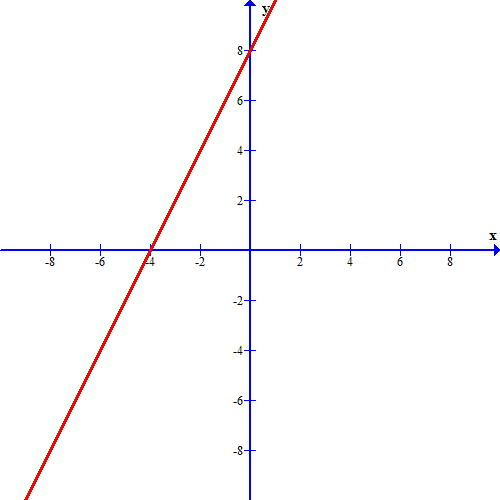#### Question 2 2. What is the solution to the system of equations shown in the graph below?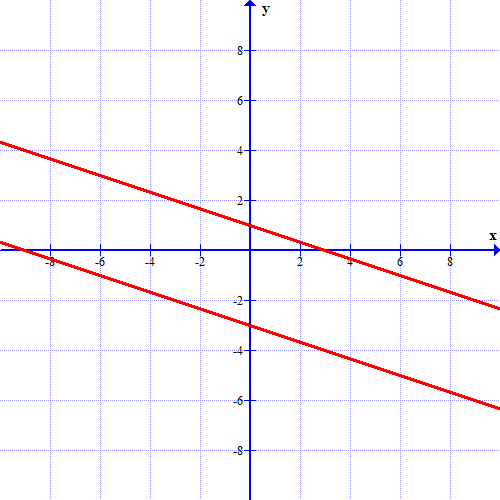#### Question 4 4. Find the equation of the line shown in the graph below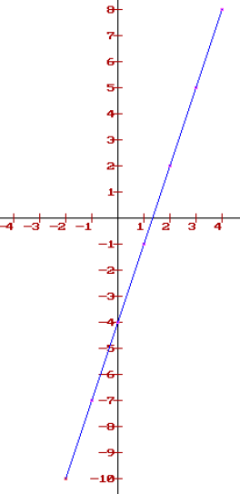### Page 4

#### Question 16 16. If the graph below has the equation Ax + By = -4, what must be true about A and B?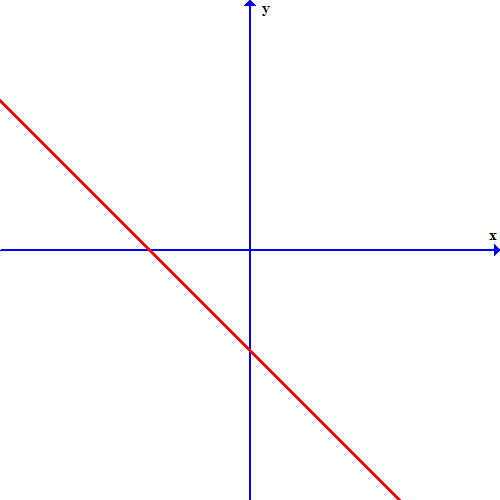#### Question 17 17. Look at the graph. What is the solution to the system of equations?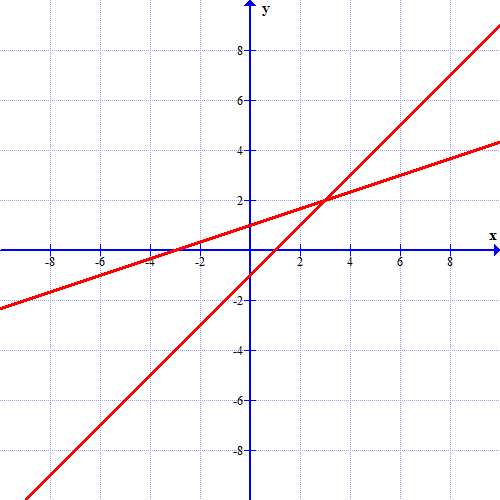### Page 6

#### Question 27 27. Which of these equations represents a line that is perpendicular to the line in the graph below?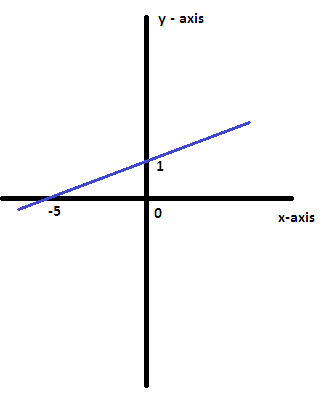#### Question 30 30. If the graph below was written in the slope intercept form, which of the following would be true?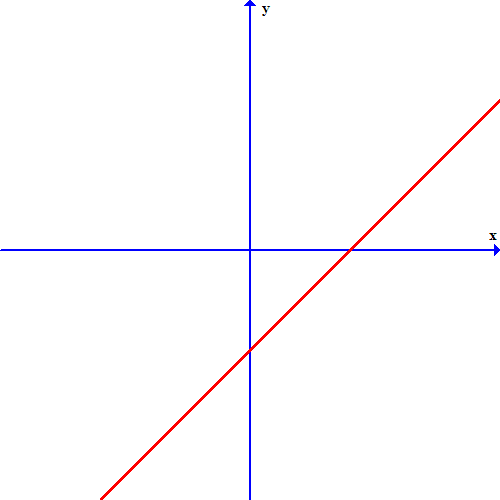#### Linear Equations Chapter Exam Instructions

Choose your answers to the questions and click 'Next' to see the next set of questions. You can skip questions if you would like and come back to them later with the yellow "Go To First Skipped Question" button. When you have completed the practice exam, a green submit button will appear. Click it to see your results. Good luck!

Support